# Mathematics - Mathematical Analysis Indefinite and Riemann Integrals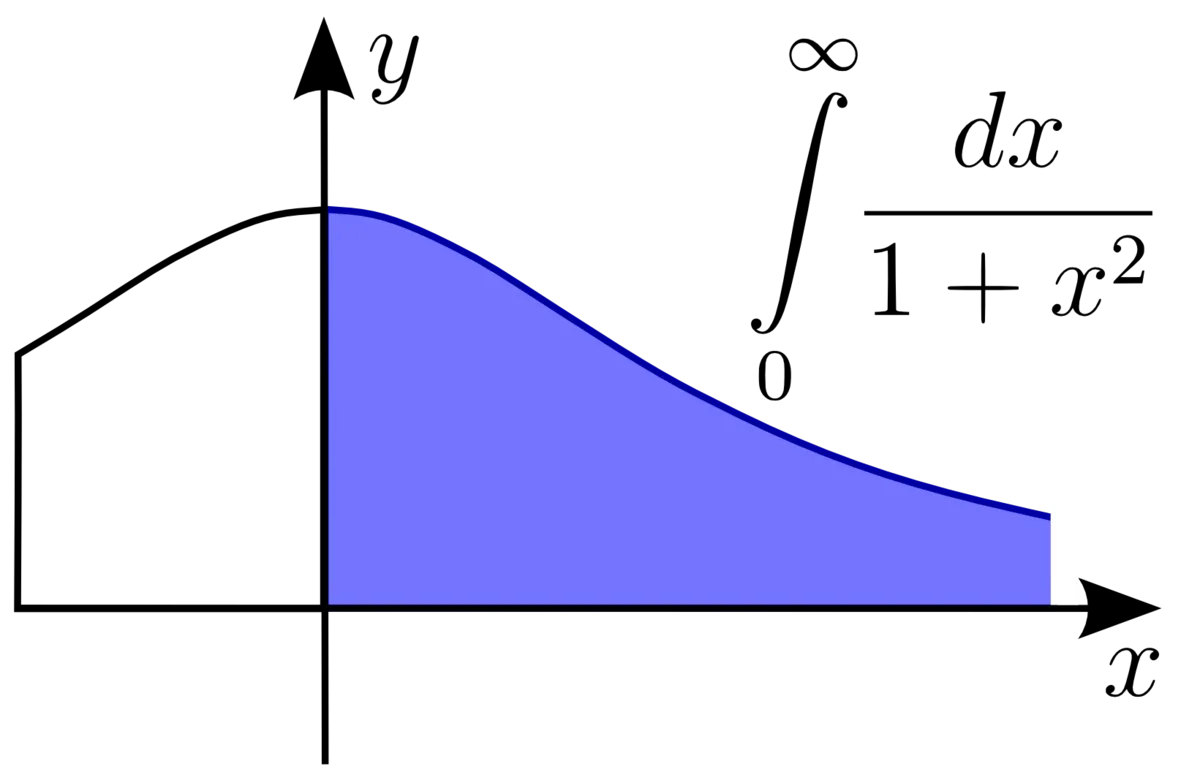Hello its a me again! Today we will continue with Mathematical Analysis getting into Integrals! I will talk about indefinite and Riemann(definite) integrals, properties etc. I will get into Integration Techniques from the next post on to talk about 1-2 each time and explain the process more in depth. Integration is the opposite of Differentiation on so I suggest you to check out the posts about Differentiation (Derivatives and Differentiation Theorems) first. So, without further do let's get started!

# Definition of an Integral:

Let's start off with a small recap. We said that a derivative's value for some specific point is equal to the slope of the tangent line at that point if we plot the graph of our funciton. So, the first derivative f'(x) from a function f gives the slope for all the points in the definition set (or domain) of our function. All this is a part of the Differential Calculus.

In the same way think about having a function g, whose values are equal to the slope of some unknown function f. Can we get a function f so that the first derivative f'(x) of that function is equal to g? Well, the answer is yes. This whole process is part of the Integral Calculus.

Example:

Think about the function g(x) = 3x^2 and that this function contains the slopes of an unknown function f.

So, f'(x) = g(x), for which f?

Here we can find this out really easily, because we already know that (x^3)' =3x^2.

This means that f(x) = x^3.

So, if for some function f there exists a function F, whose derivative is f (F' = f in a subset of R) then F is called a antiderivative, primitive function or indefinite integral. I will stick with the term integral for now.

### The constant of integration c:

If F'(x) = 0 for all the x in a range (a, b) then F(x) = c, where c is called the constant of integration.

You will see this c alot, cause there actually are more then one integral functions for a specific "slope"-derivative function given. To get the "right" one for a specific range you will have to know some specific value like f(1) = 2 so that you can eliminate the c real and put a specific real value.

There we could actually say that f(x) = x^3 + c, cause c' = 0 and so the functions:

x^3,

x^3 + 1,

x^3 + 2.56,

x^3 - 52.23

have all a derivative that equal g(x) and so are all an integral of 2x^2.

So, when there are function derivatives in an equality F'(x) = G'(x), then these two function don't need to be equal, but F(x) = G(x) + c, where c is some real value.

### Integral Definition:

Suppose a subset I in R and a function f: I -> R. The set of all the antiderivatives of f in I is called an indefinite integral. So, when F is representing the antiderivative we have that: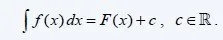where f is the function being integrated, dx is the differential of independent x and c is the constant of integration.

This giant S like thing is the way we represent integrals and you will see it alot from now on! Because, it's difficult to write it in a post I while either use some online site and make screenshots or write integral first, the same way as I did with roots.

## Linearity:

• If f, g are functions that have an indefinite integral in some subset/range I then the function af + bg, where a,b non-zero has also an indefinite integral equal to:• The same applies with more then 2 functions. With f1, f2, ..., fn being integratable in I and c1, c2, ..., cn non-zero then for c1f2 + c2f2 + ... + cnfn we have: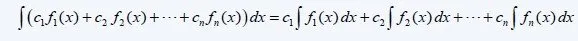Here you can find a list of all the integrals you will ever need in wikipedia. Check out only those that we covered with derivatives :)

Let's solve some examples.

### Simple Integral Examples:

1. f(x) = 5x^3 + 3e^x

From linearity we have:

integral [f(x)] = integral [5x^3] + integral [3e^x] = 5 integral [x^3] + 3 integral [e^x].

Both are very simple integrals that give us x^4/4 and e^x respectively and so:

integral [f(x)] = (5/4) x^4 + 3e^x + c (never forget c!)

2. g(x) = 4x / x^2

This is actually the case of composition f'(x)/f(x) and so:

integral [g(x)] = integral [4x/x^2] = integral [2*(x^2)'/x^2] = 2*ln(x^2), where x is non-zero

3. h(x) = arctan(x) - 5 sin(x)

This is a simple linearity and some "basic" integrals from our list and so:

integral [h(x)] = x*arctan(x) - (1/2)*ln|1+x^2| -5*(-cos(x)) + c

integral[h(x)] = x^arctan(x) - (1/2)*ln|1+x^2| + 5cos(x) + c, where c is a real number.

When the integral is not in some of those forms, we will have to do something to bring it to that form. This is exactly what we will cover from next time on...

## Definite (or Riemann) Integral:

The definite integral is used in many things, like finding the area or the volume.

Riemann's sum is based on partitions in the whole closed range [a, b] where we select random points x0, x1, ..., xn so that a = x0 < x1 <  ... < xn = b. Those subranges are [x0, x1], [x1, x2], ..., [xn-1, xn]. this whole set of subranges is called a partition of [a, b] and we can have an infinite amount of such partitions. The length of those subranges is symbolized as |Δxi| = [xi-1, xi]. Δx equal the max of those Δxi values. If Δx = b-a/n then we say that the partitioning is normal.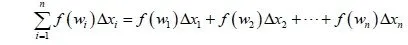whre wi is a random point in the subrange [xi-1, xi] every time.

This is Riemann's sum and if you think about the graph of a function you will see that each term of this sum is actually representing the area of an small rectangle and so the sum of all those small rectangles gives us the whole area at of f in [a, b]Suppose we let Δx go to 0 in a limit then we have: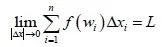, where the real number L is the area of f in [a, b].

This exact limit is called Riemann's integral and represents an definite integral.where a is the low bound of integration and b the high bound.

The following properties apply:So, the definite integral in the opposite direction has the opposite value, but the same absolute value.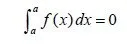The integral at some point is equal to o, because the area is equal to 0. We can also have 0 if the function is on top of axis x'x for some subrange with area L and under axis x'x for some other subrange with area -L so that the whole sum gives us L - L = 0.

So, after this last point you see that Riemann's integral gives us the area of f only when f is positive in this whole subrange, else we would have to split the range in more and subtract the ones that are negative to get the whole area of f!

## Fundamental theorem of Integral Calculus:

If a function f is integratable in a range [a, b] and F is any antiderivative of f in [a, b] then: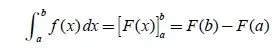This means that we have to find the indefinite integral of f that is the function F and then simply substract the value that F gives us with x = b and x = a

Example:

Let's get back to our first example and solve it for some range.

g(x) = 3x^2 and we found that f(x) = x^3 + c, where we will ignore the c part for now.

So, the integral of g(x) in some range [1, 3] is:

integral 1->3 [g(x)] = [x^3] 1->3 = 3^3 - 1^3 = 27 - 1 = 26.

## Integratable function properties:

1. A function f in [a, b] that is monotonic is also integratable in [a, b]
2. A function f in [a, b] that is continuous is also integratable in [a, b]
3. If a function f is integratable in range [a, b] than we can also integrate f in any subrange of [a, b]
4. If a function f is integratable in [a, b] and c is in (a, b) then we can split the range in two by calculating the integral of f from a to c and from c to b and summing them up.
5. If f, g are integratable in [a, b] then the lineariry applies the same way as with indefinite integrals
6. If for a function f that is integratable in [a, b] we know that f(x)>=0 then the integral in that range is also  greater than or equal to zero
7. If f, g are integratable in [a, b] and f(x) <= g(x) then the integrals have also the same relation.
8. If f is integratable in some range [a, b] then the absolute value of the integral is equal to the integral of absolute f or |f(x)|.
9. If f is an odd function in [-a, a], with a>0 then the integral in that range is equal to zero
10. If f is an even function in [-a, a], with a>0 then the integral in that range is 2 times the integral of 0 to a.

## Mean value theorem in integrals:

If f is a continuous function in [a, b] then there is some value c in [a, b] so that:The value f(c) is called the average value or mean value of f in the range [a, b].

This means that the area of f is equal to the area that x=a, x=b and x'x represent, but also equal to the area of an rectangle with b-a and f(c) length sides, for some c in [a, b]. So, L = f(c)*(b-a).

And this is actually it for today and I hope that you enjoyed it!

As I already said in the beginning of this post, I will from now on cover Integration Techniques, 1-2 each post, so that you get a better understanding of the process in each type. We will also get into some applications of integrals afterwars. When we are finished with all this stuff, I'm thinking of talking about some more difficult limits that contain roots and are in indeterminate form and then get into Sequences and Series.

Bye!

H2
H3
H4
3 columns
2 columns
1 column
1 Comment